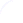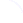Search
 Submission Procedure share: |available in:   PDF (176 kB) PS (171 kB)

get:
 Similar Docs BibTeX Write a comment
get:

DOI:   10.3217/jucs-015-03-0523

### Linear and Quadratic Complexity Bounds on the Values of the Positive Roots of Polynomials

Alkiviadis G. Akritas (University of Thessaly, Greece)

Abstract: In this paper we review the existing linear and quadratic complexity (upper) bounds on the values of the positive roots of polynomials and their impact on the performance of the Vincent-Akritas-Strzeboński (VAS) continued fractions method for the isolation of real roots of polynomials. We first present the following four linear complexity bounds (two "old" and two "new" ones, respectively): Cauchy's, (C), Kioustelidis', (K), First-Lambda, (FL) and Local-Max, (LM); we then state the quadratic complexity extensions of these four bounds, namely: CQ, KQ, FLQ, and LMQ — the second, (KQ), having being presented by Hong back in 1998. All eight bounds are derived from Theorem 5 below. The estimates computed by the quadratic complexity bounds are less than or equal to those computed by their linear complexity counterparts. Moreover, it turns out that VAS(lmq) — the VAS method implementing LMQ — is 40% faster than the original version VAS(cauchy).

Keywords: Vincent's theorem, positive roots, real root isolation, upper bounds

Categories: F.2.1, G.1.5